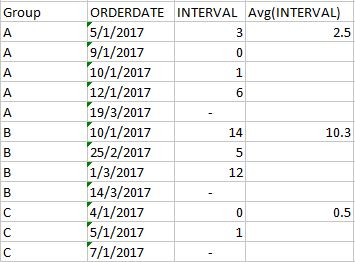QlikView App Development

Discussion Board for collaboration related to QlikView App Development.Partner

How to find the Average Interval between Days?

Hi,

I wanted to calculate the average interval days between consecutive dates. I found the interval using the following expression,

Interval(Below(date(ORDERDATE)) - date(ORDERDATE),'d')

I tried using the following expression, Aggr(Avg(Interval(Below(date(ORDERDATE)) - date(ORDERDATE),'d')))

it throws me an error in expression saying Below function is not allowed inside aggregation.

I am actually trying to get the table like,I have attached the sample file for your convenience.

Tags (2)
1 Solution

Accepted SolutionsMVP

Re: How to find the Average Interval between Days?

Try like:

Avg(total <Group> Aggr(

Interval(Below(ORDERDATE)-ORDERDATE-1,'D')

,Group,ORDERDATE))

2 RepliesMVP

Re: How to find the Average Interval between Days?

Try like:

Avg(total <Group> Aggr(

Interval(Below(ORDERDATE)-ORDERDATE-1,'D')

,Group,ORDERDATE))Partner

Re: How to find the Average Interval between Days?

Hi Tresesco,

Thanks for helping me with the expression. It works for good.